Want to share your content on R-bloggers? click here if you have a blog, or here if you don't.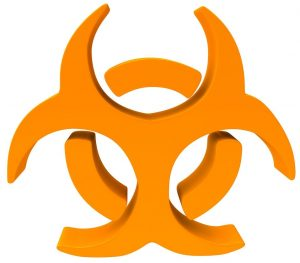A new invisible enemy, only 30kb in size, has emerged and is on a killing spree around the world: 2019-nCoV, the Novel Coronavirus!

It has already killed more people than the SARS pandemic and its outbreak has been declared a Public Health Emergency of International Concern (PHEIC) by the World Health Organization (WHO).

If you want to learn how epidemiologists estimate how contagious a new virus is and how to do it in R read on!

There are many epidemiological models around, we will use one of the simplest here, the so-called SIR model. We will use this model with the latest data from the current outbreak of 2019-nCoV (from here: Wikipedia: Case statistics). You can use the following R code as a starting point for your own experiments and estimations.

Before we start to calculate a forecast let us begin with what is confirmed so far:

Infected <- c(45, 62, 121, 198, 291, 440, 571, 830, 1287, 1975, 2744, 4515, 5974, 7711, 9692, 11791, 14380, 17205, 20440)
Day <- 1:(length(Infected))
N <- 1400000000 # population of mainland china

old <- par(mfrow = c(1, 2))
plot(Day, Infected, type ="b")
plot(Day, Infected, log = "y")
abline(lm(log10(Infected) ~ Day))
title("Confirmed Cases 2019-nCoV China", outer = TRUE, line = -2)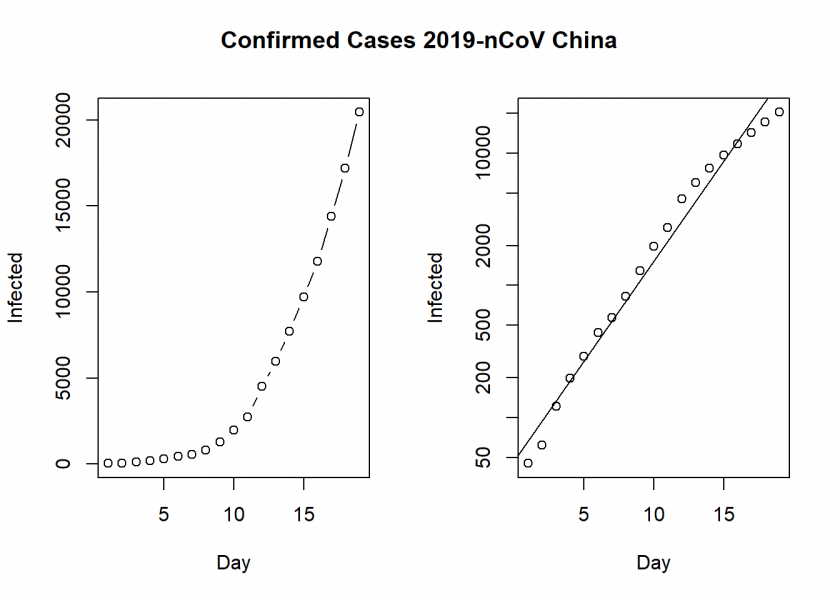On the left, we see the confirmed cases in mainland China and on the right the same but with a log scale on the y-axis (a so-called semi-log plot or more precisely log-linear plot here), which indicates that the epidemic is in an exponential phase, although at a slightly smaller rate than at the beginning. By the way: many people were not alarmed at all at the beginning. Why? Because an exponential function looks linear in the beginning. It was the same with HIV/AIDS when it first started.

Now we come to the prediction part with the SIR model, which basic idea is quite simple. There are three groups of people: those that are healthy but susceptible to the disease (), the infected () and the people who have recovered (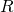):

To model the dynamics of the outbreak we need three differential equations, one for the change in each group, where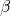is the parameter that controls the transition betweenandand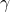which controls the transition betweenand:This can easily be put into R code:

SIR <- function(time, state, parameters) {
par <- as.list(c(state, parameters))
with(par, {
dS <- -beta/N * I * S
dI <- beta/N * I * S - gamma * I
dR <- gamma * I
list(c(dS, dI, dR))
})
}


To fit the model to the data we need two things: a solver for differential equations and an optimizer. To solve differential equations the function ode from the deSolve package (on CRAN) is an excellent choice, to optimize we will use the optim function from base R. Concretely, we will minimize the sum of the squared differences between the number of infectedat timeand the corresponding number of predicted cases by our model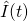: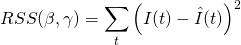Putting it all together:

library(deSolve)
init <- c(S = N-Infected, I = Infected, R = 0)
names(parameters) <- c("beta", "gamma")
out <- ode(y = init, times = Day, func = SIR, parms = parameters)
fit <- out[ , 3]
sum((Infected - fit)^2)
}

Opt <- optim(c(0.5, 0.5), RSS, method = "L-BFGS-B", lower = c(0, 0), upper = c(1, 1)) # optimize with some sensible conditions
Opt$message ##  "CONVERGENCE: REL_REDUCTION_OF_F <= FACTR*EPSMCH" Opt_par <- setNames(Opt$par, c("beta", "gamma"))
Opt_par
##      beta     gamma
## 0.6746089 0.3253912

t <- 1:70 # time in days
fit <- data.frame(ode(y = init, times = t, func = SIR, parms = Opt_par))
col <- 1:3 # colour

matplot(fit$time, fit[ , 2:4], type = "l", xlab = "Day", ylab = "Number of subjects", lwd = 2, lty = 1, col = col) matplot(fit$time, fit[ , 2:4], type = "l", xlab = "Day", ylab = "Number of subjects", lwd = 2, lty = 1, col = col, log = "y")
## Warning in xy.coords(x, y, xlabel, ylabel, log = log): 1 y value <= 0
## omitted from logarithmic plot

points(Day, Infected)
legend("bottomright", c("Susceptibles", "Infecteds", "Recovereds"), lty = 1, lwd = 2, col = col, inset = 0.05)
title("SIR model 2019-nCoV China", outer = TRUE, line = -2)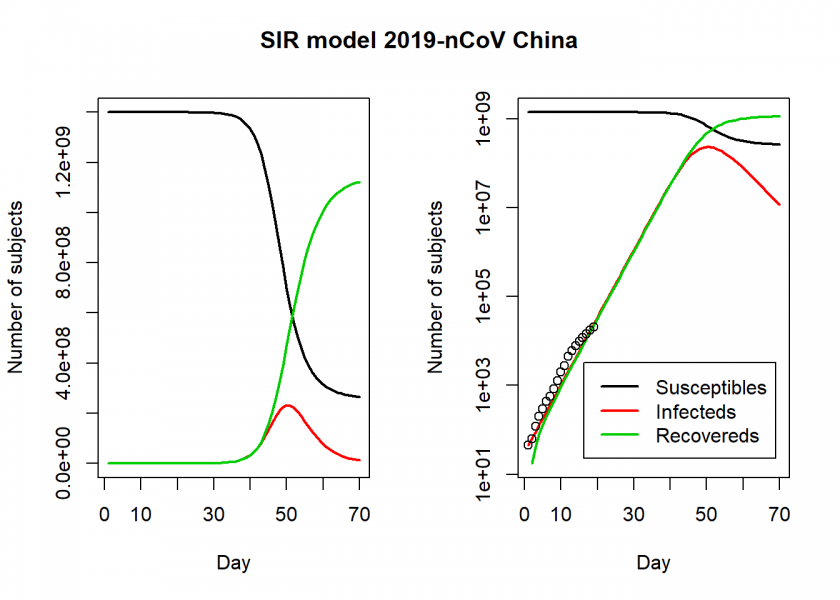We see in the right log-linear plot that the model seems to fit the values quite well. We can now extract some interesting statistics. One important number is the so-called basic reproduction number (also basic reproduction ratio)(pronounced “R naught”) which basically shows how many healthy people get infected by a sick person on average:par(old)

R0 <- setNames(Opt_par["beta"] / Opt_par["gamma"], "R0")
R0
##       R0
## 2.073224

fit[fit$I == max(fit$I), "I", drop = FALSE] # height of pandemic
##            I
## 50 232001865

max(fit\$I) * 0.02 # max deaths with supposed 2% mortality rate
##  4640037


So,is slightly above 2, which is the number many researchers and the WHO give and which is around the same range of SARS, Influenza or Ebola (while transmission of Ebola is via bodily fluids and not airborne droplets). Additionally, according to this model, the height of a possible pandemic would be reached by the beginning of March (50 days after it started) with over 200 million Chinese infected and over 4 million dead!

Do not panic! All of this is preliminary and hopefully (probably!) false. When you play along with the above model you will see that the fitted parameters are far from stable. On the one hand, the purpose of this post was just to give an illustration of how such analyses are done in general with a very simple (probably too simple!) model, on the other hand, we are in good company here; the renowned scientific journal nature writes:

Researchers are struggling to accurately model the outbreak and predict how it might unfold.

On the other hand, I wouldn’t go that far that the numbers are impossibly high. H1N1, also known as swine flu, infected up to 1.5 billion people during 2009/2010 and nearly 600,000 died. And this wasn’t the first pandemic of this proportion in history (think Spanish flu). Yet, this is one of the few times where I hope that my model is wrong and we will all stay healthy!